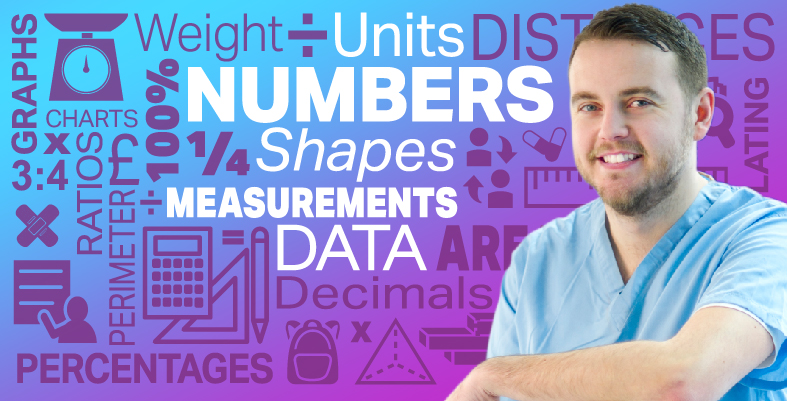Everyday maths for Health and Social Care and Education Support 1

Start this free course now. Just create an account and sign in. Enrol and complete the course for a free statement of participation or digital badge if available.

Free course

# 9.2 Function machinesFigure 28 A function machine

Function machines can help when you are working with any formula that has more than one step. The difference between formulas and function machines is that you must follow a function machine in the correct order from left to right, or top to bottom, as shown in the example below. In the Level 2 course on maths you will see that when you use formulas, the BIDMAS rule must be followed.

## Example: Marathon training

Dominic wants to run a marathon in under four hours. He finds the following method to work out his expected marathon time: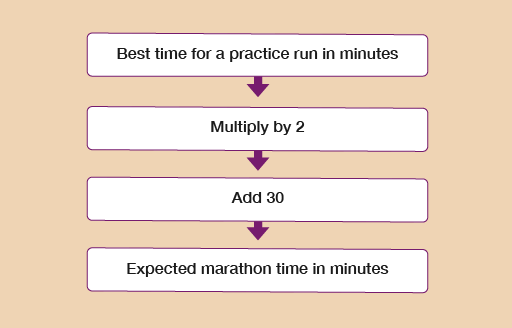Figure 29 A function machine flow chart

Dominic’s best time for a practice run is 98 minutes. If he runs a marathon at the same pace, will he complete it in less than four hours?

### Method

Dominic’s best practice run is 98 minutes, so you need to put 98 as the first number in the function machine:

98 × 2 + 30 = expected marathon time

98 × 2 + 30 = 226 minutes

So to answer the question: yes, if Dominic runs a full marathon at the same pace he runs during practice, he would finish the marathon in under four hours.

Now you have read the example, please have a go at the following activity.

## Activity 22: Using function machines

1. The battle of the bands will take place in the youth club hall.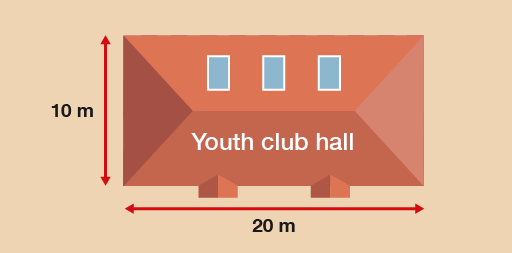Figure 30 A youth club

Shazad uses the following rule to find out the number of people allowed in any hall: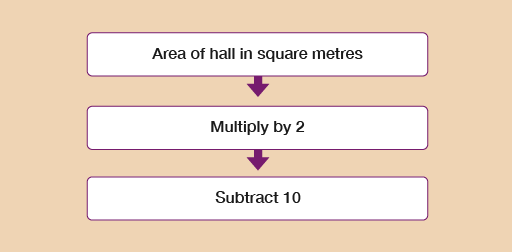Figure 31 A function machine flow chart

What is the number of people allowed in the youth club hall?

If you were to write the function machine as a formula, it would look like this:

• (Area of hall in square metres × 2) – 10 = number of people allowed in the youth club hall

The area of the hall in square metres is:

• 10 × 20 = 200 m²

So we would replace ‘Area of hall in square metres’ in the formula with 200:

• (200 × 2) – 10 = 390

So the maximum number of people allowed in the youth club hall is 390 people.

1. Simon meets a trainer at the leisure centre to set fitness goals. The trainer uses the following rule to calculate Simon’s BMI:

• Simon’s weight in kg ÷ 3 = Simon’s BMI

One of Simon’s fitness goals is to have a BMI between 19 and 25.

He currently weighs 72 kg. Is he meeting his fitness goal?

Simon’s weight is 72 kg so the calculation is:

• 72 ÷ 3 = 24

Simon’s BMI is 24, so he has met his fitness goal.

1. The flow chart below is used to work out the correct medicine dosage for a child: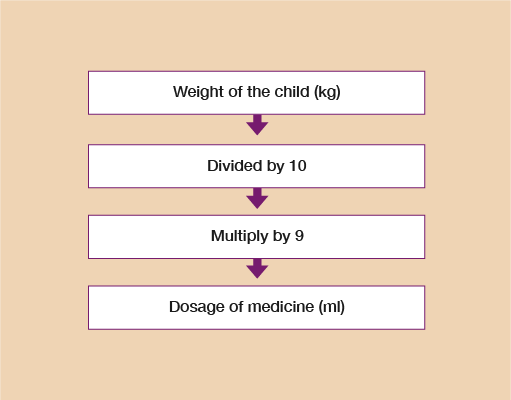Figure 32 A function machine flow chart

Lena weighs 40kg. Work out the correct dosage of medicine for Lena

If you were to write the medicine dosage as a formula, it would look like this:

• (Patient's weight in kg ÷ 10) × 9 = dosage of medicine in ml

Lena weighs 40 kg, so we would replace ‘Patient's weight in kg’ in the formula with 40:

• (40 ÷ 10) × 9 = 36

So the correct dosage of medicine for Lena is 36 ml.

1. The flow chart below is used to calculate the number of staff needed each day at a children’s nursery:

Kofi uses this rule to work out how many staff are required each day:Figure 33 A function machine flow chart

On a Monday, 48 children attend the nursery. Kofi thinks that he needs 6 members of staff, is this correct?

If you were to write the function machine as a formula, it would look like this:

• (Number of children ÷ 10) rounded to the nearest whole number + 2 = number of staff needed

48 children attend the nursery on a Monday, so we would replace ‘Number of children’ in the formula with 48:

• (48 ÷ 10) = 5 (rounded to the nearest whole number) + 2 = 7

So Kofi is wrong. The number of staff needed is 7 not 6.

You have now completed the section on working with word formulas and function machines. If you did not get the questions correct, please return to them and identify where you went wrong.

## Summary

In this section you have learned about working with word formulas and function machines.

FSM_SSH_1